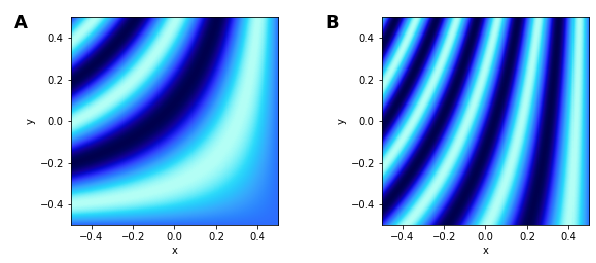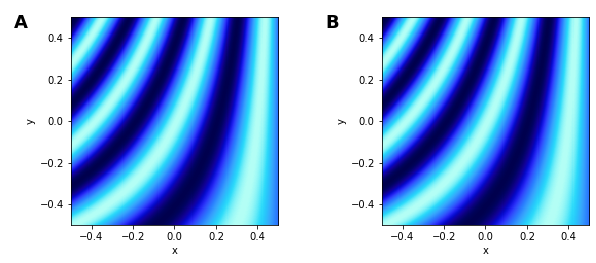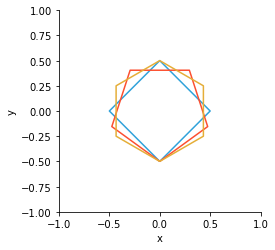# Live Data#

The HoloMap is a core HoloViews data structure that allows easy exploration of parameter spaces. The essence of a HoloMap is that it contains a collection of Elements (e.g. `Image`s and `Curve`s) that you can easily select and visualize.

HoloMaps hold fully constructed Elements at specifically sampled points in a multidimensional space. Although HoloMaps are useful for exploring high-dimensional parameter spaces, they can very quickly consume huge amounts of memory to store all these Elements. For instance, a hundred samples along four orthogonal dimensions would need a HoloMap containing a hundred million Elements, each of which could be a substantial object that takes time to create and costs memory to store. Thus `HoloMaps` have some clear limitations:

• HoloMaps may require the generation of millions of Elements before the first element can be viewed.

• HoloMaps can easily exhaust all the memory available to Python.

• HoloMaps can even more easily exhaust all the memory in the browser when displayed.

• Static export of a notebook containing HoloMaps can result in impractically large HTML files.

The `DynamicMap` addresses these issues by computing and displaying elements dynamically, allowing exploration of much larger datasets:

• DynamicMaps generate elements on the fly, allowing the process of exploration to begin immediately.

• DynamicMaps do not require fixed sampling, allowing exploration of parameters with arbitrary resolution.

• DynamicMaps are lazy in the sense they only compute as much data as the user wishes to explore.

Of course, these advantages come with some limitations:

• DynamicMaps require a live notebook server and cannot be fully exported to static HTML.

• DynamicMaps store only a portion of the underlying data, in the form of an Element cache and their output is dependent on the particular version of the executed code.

• DynamicMaps (and particularly their element caches) are typically stateful (with values that depend on patterns of user interaction), which can make them more difficult to reason about.

In addition to the different computational requirements of `DynamicMaps`, they can be used to build sophisticated, interactive visualisations that cannot be achieved using only `HoloMaps`. This notebook demonstrates some basic examples and the Responding to Events guide follows on by introducing the streams system. The Custom Interactivity shows how you can directly interact with your plots when using the Bokeh backend.

When DynamicMap was introduced in version 1.6, it supported multiple different ‘modes’ which have now been deprecated. This notebook demonstrates the simpler, more flexible and more powerful DynamicMap introduced in version 1.7. Users who have been using the previous version of DynamicMap should be unaffected as backwards compatibility has been preserved for the most common cases.

All this will make much more sense once we’ve tried out some `DynamicMaps` and showed how they work, so let’s create one!

## `DynamicMap`#

```import numpy as np
import holoviews as hv
from holoviews import opts

hv.extension('matplotlib')
```We will now create `DynamicMap` similar to the `HoloMap` introduced in the Introductory guide. The `HoloMap` in that introduction consisted of `Image` elements defined by a function returning NumPy arrays called `sine_array`. Here we will define a `waves_image` function that returns an array pattern parameterized by arbitrary `alpha` and `beta` parameters inside a HoloViews `Image` element:

```xvals  = np.linspace(-4, 0, 202)
yvals  = np.linspace(4, 0, 202)
xs, ys = np.meshgrid(xvals, yvals)

def waves_image(alpha, beta):
return hv.Image(np.sin(((ys/alpha)**alpha+beta)*xs))

waves_image(1,0) + waves_image(1,4)
```Now we can demonstrate the possibilities for exploration enabled by the simplest declaration of a `DynamicMap`.

### Basic `DynamicMap` declaration#

A simple `DynamicMap` declaration looks identical to that needed to declare a `HoloMap`. Instead of supplying some initial data, we will supply the `waves_image` function with key dimensions simply declaring the arguments of that function:

```dmap = hv.DynamicMap(waves_image, kdims=['alpha', 'beta'])
dmap
```
```DynamicMap cannot be displayed without explicit indexing as 'alpha', 'beta' dimension(s) are unbounded.
Set dimensions bounds with the DynamicMap redim.range or redim.values methods.
```
```:DynamicMap   [alpha,beta]
```

This object is created instantly, but because it doesn’t generate any `hv.Image` objects initially it only shows the printed representation of this object along with some information about how to display it. We will refer to a `DynamicMap` that doesn’t have enough information to display itself as ‘unbounded’.

The textual representation of all `DynamicMaps` look similar, differing only in the listed dimensions until they have been evaluated at least once.

#### Explicit indexing#

Unlike a corresponding `HoloMap` declaration, this simple unbounded `DynamicMap` cannot yet visualize itself. To view it, we can follow the advice in the warning message. First we will explicitly index into our `DynamicMap` in the same way you would access a key on a `HoloMap`:

```dmap[1,2] + dmap.select(alpha=1, beta=2)
```Note that the declared kdims are specifying the arguments by position as they do not match the argument names of the `waves_image` function. If you do match the argument names exactly, you can map a kdim position to any argument position of the callable. For instance, the declaration `kdims=['freq', 'phase']` would index first by frequency, then phase without mixing up the arguments to `waves_image` when indexing.

#### Setting dimension ranges#

The second suggestion in the warning message was to supply dimension ranges using the `redim.range` method:

```dmap.redim.range(alpha=(1, 5.0), beta=(1, 6.0))
```

Here each `hv.Image` object visualizing a particular sine ring pattern with the given parameters is created dynamically, whenever the slider is set to a new value. Any value in the allowable range can be requested by dragging the sliders or by tweaking the values using the left and right arrow keys.

Of course, we didn’t have to use the `redim.range` method and we could have simply declared the ranges right away using explicit `hv.Dimension` objects. This would allow us to declare other dimension properties such as the step size used by the sliders: by default each slider can select around a thousand distinct values along its range but you can specify your own step value via the dimension `step` parameter. If you use integers in your range declarations, integer stepping will be assumed with a step size of one.

Note that whenever the `redim` method is used, a new `DynamicMap` is returned with the updated dimensions. In other words, the original `dmap` remains unbounded with default dimension objects.

#### Setting dimension values#

The `DynamicMap` above allows exploration of any phase and frequency within the declared range unlike an equivalent `HoloMap` which would have to be composed of a finite set of samples. We can achieve a similar discrete sampling using `DynamicMap` by setting the `values` parameter on the dimensions:

```dmap.redim.values(alpha=[1, 2, 3], beta=[0.1, 1.0, 2.5])
```

The sliders now snap to the specified dimension values and if you are running this live, the above cell should look like a HoloMap. `DynamicMap` is in fact a subclass of `HoloMap` with some crucial differences:

• You can now pick as many values of alpha or beta as allowed by the slider.

• What you see in the cell above will not be exported in any HTML snapshot of the notebook

#### Getting the current key#

The `current_key` property returns the current key of the `DynamicMap`, i.e. either a value (one-dimensional) or a tuple (multi-dimensional) that represents the current state of the widgets. If you move the sliders above and re-execute the cell below you will see that the tuple returned reflects the values of alpha and beta.

```dmap.current_key
```
```(1, 2.5)
```

We will now explore how `DynamicMaps` relate to `HoloMaps` including conversion operations between the two types. As we will see, there are other ways to display a `DynamicMap` without using explicit indexing or redim.

## Interaction with `HoloMap`s#

To explore the relationship between `DynamicMap` and `HoloMap`, let’s declare another callable to draw some shapes we will use in a new `DynamicMap`:

```def shapes(N, radius=0.5): # Positional keyword arguments are fine
for a in np.linspace(-np.pi, np.pi, n+2)]],
extents=(-1,-1,1,1))
for n in range(N,N+3)]
return hv.Overlay(paths)
```

### Sampling `DynamicMap` from a `HoloMap`#

When combining a `HoloMap` with a `DynamicMap`, it would be very awkward to have to match the declared dimension `values` of the DynamicMap with the keys of the `HoloMap`. Fortunately you don’t have to:

```holomap = hv.HoloMap({(N,r):shapes(N, r) for N in [3,4,5] for r in [0.5,0.75]},  kdims=['N', 'radius'])
holomap + dmap
```

Here we declared a `DynamicMap` without using `redim`, but we can view its output because it is presented alongside a `HoloMap` which defines the available keys. This convenience is subject to three particular restrictions:

• You cannot display a layout consisting of unbounded `DynamicMaps` only, because at least one HoloMap is needed to define the samples.

• The HoloMaps provide the necessary information required to sample the DynamicMap.

Note that there is one way `DynamicMap` is less restricted than `HoloMap`: you can freely combine bounded `DynamicMaps` together in a `Layout`, even if they don’t share key dimensions.

### Converting from `DynamicMap` to `HoloMap`#

Above we mentioned that `DynamicMap` is an instance of `HoloMap`. Does this mean it has a `.data` attribute?

```dtype = type(dmap.data).__name__
length = len(dmap.data)
print("DynamicMap 'dmap' has an {dtype} .data attribute of length {length}".format(dtype=dtype, length=length))
```
```DynamicMap 'dmap' has an dict .data attribute of length 1
```

This is exactly the same sort of `.data` as the equivalent `HoloMap`, except that its values will vary according to how much you explored the parameter space of `dmap` using the sliders above. In a `HoloMap`, `.data` contains a defined sampling along the different dimensions, whereas in a `DynamicMap`, the `.data` is simply the cache.

The cache serves two purposes:

• Avoids recomputation of an element should we revisit a particular point in the parameter space. This works well for categorical or integer dimensions, but doesn’t help much when using continuous sliders for real-valued dimensions.

• Records the space that has been explored with the `DynamicMap` for any later conversion to a `HoloMap` up to the allowed cache size.

We can always convert any `DynamicMap` directly to a `HoloMap` as follows:

```hv.HoloMap(dmap)
```This is in fact equivalent to declaring a HoloMap with the same parameters (dimensions, etc.) using `dmap.data` as input, but is more convenient. Note that the slider positions reflect those we sampled from the `HoloMap` in the previous section.

Although creating a HoloMap this way is easy, the result is poorly controlled, as the keys in the DynamicMap cache are usually defined by how you moved the sliders around. If you instead want to specify a specific set of samples, you can easily do so by using the same key-selection semantics as for a `HoloMap` to define exactly which elements are to be sampled and put into the cache:

```hv.HoloMap(dmap[{(2,0.3), (2,0.6), (3,0.3), (3,0.6)}])
```

Here we index the `dmap` with specified keys to return a new DynamicMap with those keys in its cache, which we then cast to a `HoloMap`. This allows us to export specific contents of `DynamicMap` to static HTML which will display the data at the sampled slider positions.

The key selection above happens to define a Cartesian product, which is one of the most common ways to sample across dimensions. Because the list of such dimension values can quickly get very large when enumerated as above, we provide a way to specify a Cartesian product directly, which also works with `HoloMaps`. Here is an equivalent way of defining the same set of four points in that two-dimensional space:

```samples = hv.HoloMap(dmap[{2,3},{0.5,1.0}])
samples
```
```samples.data.keys()
```
```dict_keys([(2, 0.5), (2, 1.0), (3, 0.5), (3, 1.0)])
```

The default cache size of 500 Elements is relatively high so that interactive exploration will work smoothly, but you can reduce it using the `cache_size` parameter if you find you are running into issues with memory consumption. A bounded `DynamicMap` with `cache_size=1` requires the least memory, but will recompute a new Element every time the sliders are moved, making it less responsive.

### Converting from `HoloMap` to `DynamicMap`#

We have now seen how to convert from a `DynamicMap` to a `HoloMap` for the purposes of static export, but why would you ever want to do the inverse?

Although having a `HoloMap` to start with means it will not save you memory, converting to a `DynamicMap` does mean that the rendering process can be deferred until a new slider value requests an update. You can achieve this conversion using the `Dynamic` utility as demonstrated here by applying it to the previously defined `HoloMap` called `samples`:

```from holoviews.util import Dynamic
dynamic = Dynamic(samples)
print('After apply Dynamic, the type is a {dtype}'.format(dtype=type(dynamic).__name__))
dynamic
```
```After apply Dynamic, the type is a DynamicMap
```

In this particular example, there is no real need to use `Dynamic` as each frame renders quickly enough. For visualizations that are slow to render, using `Dynamic` can result in more responsive visualizations.

The `Dynamic` utility is very versatile and is discussed in more detail in the Transforming Elements guide.

### Slicing `DynamicMaps`#

As we have seen we can either declare dimension ranges directly in the kdims or use the `redim.range` convenience method:

```dmap = hv.DynamicMap(shapes, kdims=['N','radius']).redim.range(N=(2,20), radius=(0.5,1.0))
```

The declared dimension ranges define the absolute limits allowed for exploration in this continuous, bounded DynamicMap . That said, you can use the soft_range parameter to view subregions within that range. Setting the soft_range parameter on dimensions can be done conveniently using slicing:

```sliced = dmap[4:8, :]
sliced
```

Notice that N is now restricted to the range 4:8. Open slices are used to release any `soft_range` values, which resets the limits back to those defined by the full range:

```sliced[:, 0.8:1.0]
```

The `[:]` slice leaves the soft_range values alone and can be used as a convenient way to clone a `DynamicMap`. Note that mixing slices with any other object type is not supported. In other words, once you use a single slice, you can only use slices in that indexing operation.

## Using groupby to discretize a DynamicMap#

A DynamicMap also makes it easy to partially or completely discretize a function to evaluate in a complex plot. By grouping over specific dimensions that define a fixed sampling via the Dimension values parameter, the DynamicMap can be viewed as a `GridSpace`, `NdLayout`, or `NdOverlay`. If a dimension specifies only a continuous range it can’t be grouped over, but it may still be explored using the widgets. This means we can plot partial or completely discretized views of a parameter space easily.

### Partially discretize#

The implementation for all the groupby operations uses the `.groupby` method internally, but we also provide three higher-level convenience methods to group dimensions into an `NdOverlay` (`.overlay`), `GridSpace` (`.grid`), or `NdLayout` (`.layout`).

Here we will evaluate a simple sine function with three dimensions, the phase, frequency, and amplitude. We assign the frequency and amplitude discrete samples, while defining a continuous range for the phase:

```xs = np.linspace(0, 2*np.pi,100)

def sin(ph, f, amp):
return hv.Curve((xs, np.sin(xs*f+ph)*amp))

kdims=[hv.Dimension('phase', range=(0, np.pi)),
hv.Dimension('frequency', values=[0.1, 1, 2, 5, 10]),
hv.Dimension('amplitude', values=[0.5, 5, 10])]

waves_dmap = hv.DynamicMap(sin, kdims=kdims)
```

Next we define the amplitude dimension to be overlaid and the frequency dimension to be gridded:

```wave_grid = waves_dmap.overlay('amplitude').grid('frequency')
wave_grid.opts(fig_size=200, show_legend=True)
```

As you can see, instead of having three sliders (one per dimension), we’ve now laid out the frequency dimension as a discrete set of values in a grid, and the amplitude dimension as a discrete set of values in an overlay, leaving one slider for the remaining dimension (phase). This approach can help you visualize a large, multi-dimensional space efficiently, with full control over how each dimension is made visible.

### Fully discretize#

Given a continuous function defined over a space, we could sample it manually, but here we’ll look at an example of evaluating it using the groupby method. Let’s look at a spiral function with a frequency and first- and second-order phase terms. Then we define the dimension values for all the parameters and declare the DynamicMap:

```opts.defaults(opts.Path(color=hv.Palette('Blues')))

def spiral_equation(f, ph, ph2):
r = np.arange(0, 1, 0.005)
xs, ys = (r * fn(f*np.pi*np.sin(r+ph)+ph2) for fn in (np.cos, np.sin))
return hv.Path((xs, ys))

spiral_dmap = hv.DynamicMap(spiral_equation, kdims=['f','ph','ph2'])
spiral_dmap = spiral_dmap.redim.values(
f=np.linspace(1, 10, 10),
ph=np.linspace(0, np.pi, 10),
ph2=np.linspace(0, np.pi, 4))
```

Now we can make use of the `.groupby` method to group over the frequency and phase dimensions, which we will display as part of a GridSpace by setting the `container_type`. This leaves the second phase variable, which we assign to an NdOverlay by setting the `group_type`:

```spiral_grid = spiral_dmap.groupby(['f', 'ph'], group_type=hv.NdOverlay, container_type=hv.GridSpace)
spiral_grid.opts(
opts.GridSpace(xaxis=None, yaxis=None),
opts.Path(bgcolor='white', xaxis=None, yaxis=None))
```